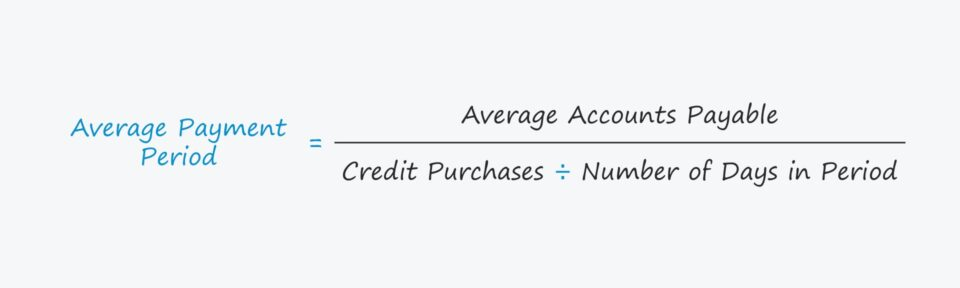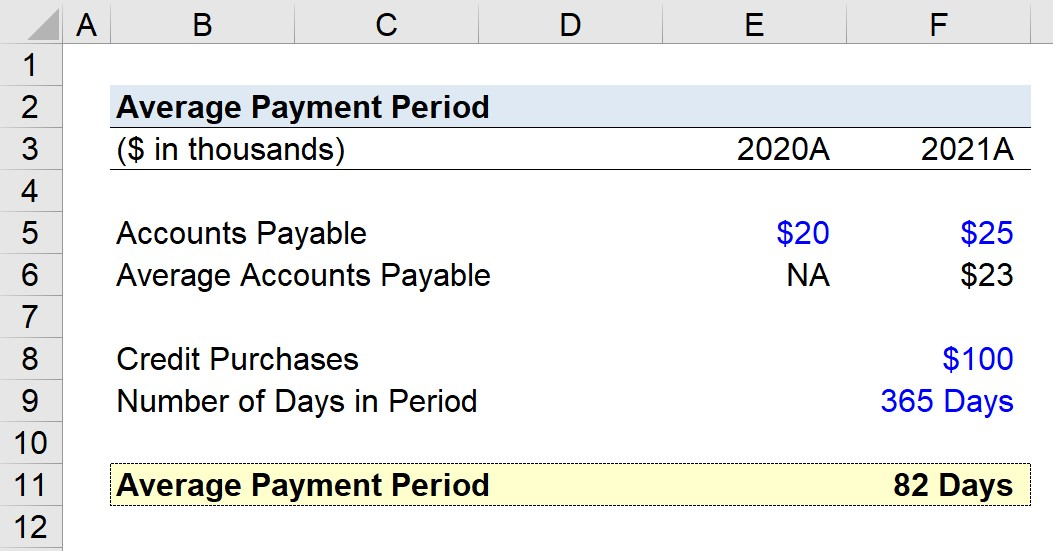Welcome to Wall Street Prep! Use code at checkout for 15% off.# Average Payment Period

Guide to Understanding the Average Payment Period## How to Calculate Average Payment Period?

The average payment period counts the number of days it takes a company, on average, to pay off its outstanding supplier or vendor invoices.

For accounts payable to be recognized on the balance sheet, the product or service was delivered to the company as part of the agreement with the supplier.

However, the company has yet to pay the related invoice, so the cash remains under the possession of the company until issued.

Until the company pays the supplier – in the form of cash (“cash outflow”) – the outstanding balance is recognized in the accounts payable (A/P) line item on its balance sheet.

• Accounts Payable (A/P) → The amount of unpaid bills still owed to suppliers/vendors for products or services already received, yet were paid for using credit, instead of cash.

While the supplier or vendor delivered the purchased good or service, the company placed the order using credit as the form of payment (and the related invoice has not yet been processed in cash).

Calculating the average payment period can be broken into a three-step process:

1. The first step is to calculate the average accounts payable by adding the end of period and beginning of period accounts payable balances, and then dividing by two.
2. The next step is to divide the dollar amount of credit purchases made by the company (i.e. orders placed using credit) and the number of days in the period (i.e. annual = 365 days).
3. In the final step, the average accounts payable balance is divided by the resulting figure from step 2 (i.e. credit purchases divided by the number of days in the period) to calculate the implied average payment period.

## Average Payment Period Formula

The formula for calculating the average payment period is as follows.

Average Payment Period = Average Accounts Payable ÷ (Credit Purchases ÷ Number of Days in Period)

Where:

1. Accounts Payable → The accounts payable line item appears on the balance sheet as a current liability and represents the accumulated balance of unpaid invoices owed to suppliers and/or vendors.
2. Number of Days in Period → The number of days in the chosen accounting period, e.g. an annual calculation, would use 365 days, or 360 days.
3. Credit Purchases → The total value of orders placed by the company on credit, not cash.

The equation to compute the average accounts payable of a company is as follows.

Average Accounts Payable = (Beginning Accounts Payable + Ending Accounts Payable) ÷ 2

So, why is the “average” accounts payable balance used?

The reason for using the average balance, rather than the ending balance, is to ensure consistency in the timing of the ratio.

In other words, the credit purchases are measured across the fiscal period, so the average accounts payable balance is usually used.

However, the ending A/P balance is often acceptable in practice, as the insights derived will rarely be that different under either approach, barring unusual circumstances.

## What is a Good Average Payment Period?

In general, the more a supplier relies on a customer, the more negotiating leverage the buyer has in terms of payment periods.

The time between the initial purchase date and the date of actual cash payment (and receipt by the supplier) is oftentimes used as a proxy for a buyer’s bargaining power, i.e. the capability of a company to exert pressure when negotiating terms with its suppliers to receive favorable terms, such as price reductions and extensions of payment due dates.

• Shorter Average Payment Period ➝ Low Bargaining Leverage (and Less Free Cash Flow)
• Longer Average Payment Period ➝ High Bargaining Leverage (and More Free Cash Flow)

Companies with more buying power and negotiating leverage typically have the following characteristics:

• Significant Order Size (or Volume)
• High Frequency of Orders (i.e. Source of Recurring Revenue)
• Long-Term Relationship with Supplier (i.e. Accumulated “Trust” over Time)
• Customer Concentration Risk (i.e. Percentage of Revenue Attributable to Single Customer)
• Niche Technical Materials (i.e. Limited Number of Potential Customers)

## Average Payment Period Calculator

We’ll now move to a modeling exercise, which you can access by filling out the form below.#### Get the Excel Template!Submitting...

## Average Payment Period Calculation Example

Suppose we’re tasked with calculating the average payment period of a company with an ending accounts payable balance of \$20k and \$25k in 2020 and 2021, respectively.

• Beginning Accounts Payable = \$20k
• Ending Accounts Payable = \$25k

Given those two values, the average accounts payable is roughly \$23k.

• Average Accounts Payable = (\$25k + \$20k) ÷ 2 = \$23k

Furthermore, we’ll assume our company made \$100k in credit purchases in fiscal year 2021.

• Credit Purchases = \$100k

Since all of our figures so far are on an annual basis, the correct number of days in the accounting period to use in our calculation is 365 days.

• Number of Days in Period = 365 Days

In closing, the average payment period for our hypothetical company is approximately 82 days, which we calculated using the formula below.

• Average Payment Period = \$23k ÷ (\$100k ÷ 365) = 82 DaysStep-by-Step Online Course

### Everything You Need To Master Financial Modeling

Enroll in The Premium Package: Learn Financial Statement Modeling, DCF, M&A, LBO and Comps. The same training program used at top investment banks.